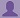SHARE
HELP

To start with, Sr2Jr’s first step is to reduce the expenses related to education. To achieve this goal Sr2Jr organized the textbook’s question and answers. Sr2Jr is community based and need your support to fill the question and answers. The question and answers posted will be available free of cost to all.# Computer Networking : A Top-down Approach

Authors:
James F. Kurose, Keith W. Ross
Exercise:
Problems
Chapter:
Computer Networks And The Internet
Edition:
6
ISBN:
9780132856201
Question:
25
 Previous Next

###Question

Suppose two hosts, A and B, are separated by 20,000 kilometers and are connected by a direct link of R = 2 Mbps. Suppose the propagation speed over the link is 2.5 x 10^8 meters/sec.

a. Calculate the bandwidth-delay product, R _ dprop.

b. Consider sending a file of 800,000 bits from Host A to Host B. Suppose the file is sent continuously as one large message. What is the maximum number of bits that will be in the link at any given time?

c. Provide an interpretation of the bandwidth-delay product.

d. What is the width (in meters) of a bit in the link? Is it longer than a football field?

e. Derive a general expression for the width of a bit in terms of the propagation speed s, the transmission rate R, and the length of the link m.

###Answer

The distance (Distance) between two hosts A and B = 20,000 km

a)

The distance (Distance) between two hosts A and B = 20,000 km

Trasmission rate(R) of the direct link between A and B =2Mbps

Propagation Speed(S) of the link between A and B

Calculate the propagation delay:

Calculate the band-width delay product:

Therefore, band-with delay product is 160000bits

b)

Size of the file =800000 bits

Trasmission rate(R) of the direct link between A and B =2Mbps

The band-width delay product:

Therefore, the maximum number of bits at a given time will be 160000bits.

c)

The product of band-windth delay is equal to the maximum number of bits on the transmission line.

d)

Trasmission rate(R) of the direct link between A and B =2Mbps

Propagation Speed(S) of the link between A and B

Formula to calculate the length of 1 bit on the transmission line

Therefore, it is longer than a football field.

e)

A general expression for the width = (Transmission rate(R) * Speed(s) )/ length of the link (m)

### Discussions

Post the discussion to improve the above solution.